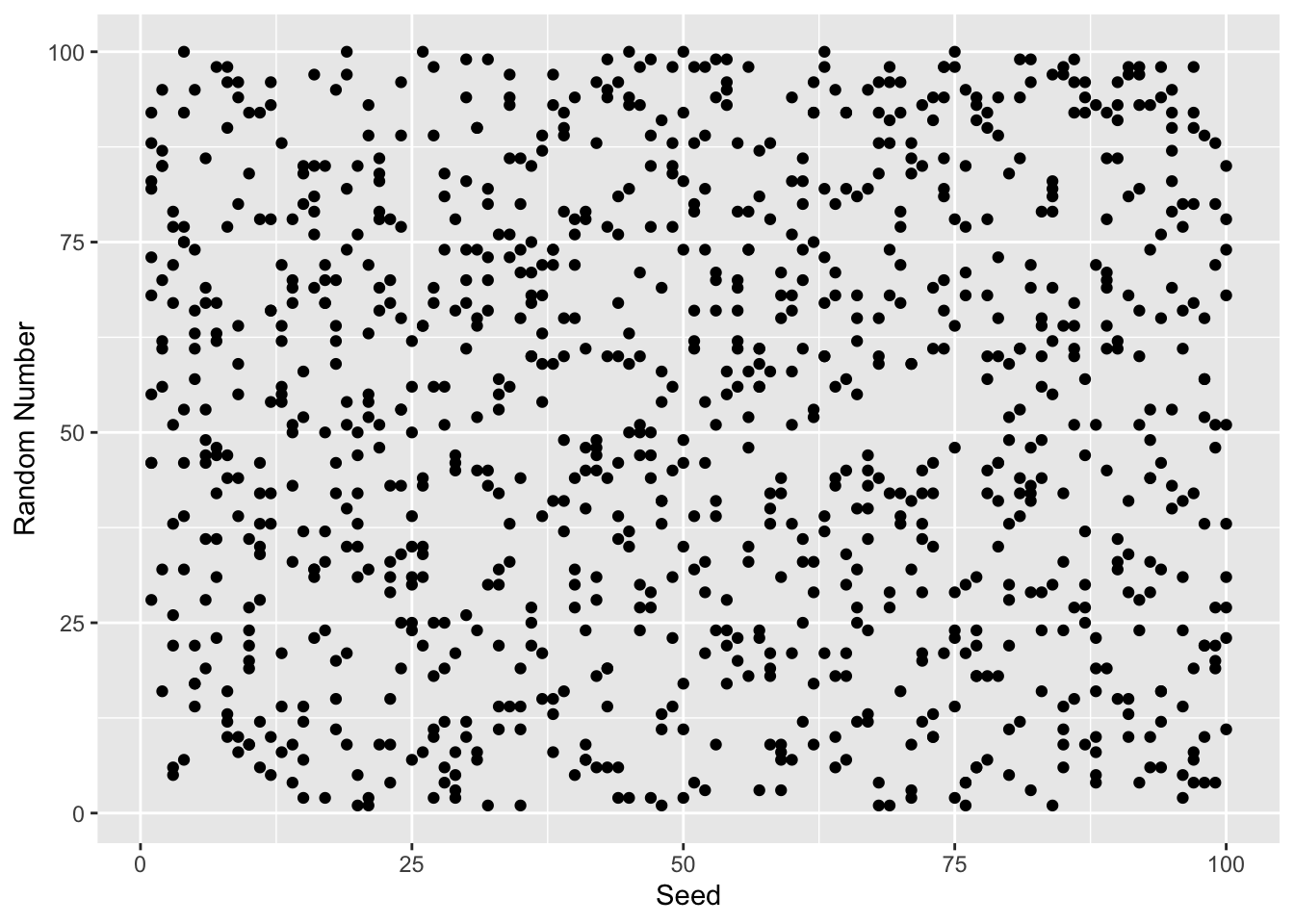# Random Numbers and Seeds in R

To explore how R generates random numbers, we will use the rnorm function. This function draws a random number from a normal distribution with a mean = 0 and standard deviation = 1 (though these can be changed with the mean and sd parameters). With n = 1 we will get one random number.

rnorm(n = 1)
##  0.6308552
rnorm(n = 1)
##  1.36274

Each time you run the command you will get a different number. The set.seed function will sets a seed to the random number generator so that each subsequent run will produce the same number.

set.seed(2112); rnorm(n = 1)
##  0.9243372
set.seed(2112); rnorm(n = 1)
##  0.9243372

Setting a different seed results in a different number.

set.seed(2113); rnorm(n = 1)
##  0.5499032

What are seeds?

Computers are actually bad at random events. However, there are good algorithms that mimic random processes (hence psydo random). These algorithms work by starting with some initial value, a seed, and executing a complex algorithm that approximates randomization. The seed is often set to the current time in milliseconds. To visualize the random process, we will use the sample function to randomly select a number between 1 and 100. We will consider the ouput for the first 1,000 seeds.

random_numbers <- integer(1000)
for(i in seq_len(length(random_numbers))) {
set.seed(i)
random_numbers[i] <- sample(1:100, size = 1)
}
library(ggplot2)
ggplot(data.frame(x = 1:100, y = random_numbers),
aes(x = x, y = y)) +
geom_point() +
xlab('Seed') + ylab('Random Number')